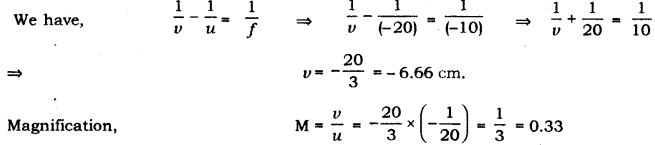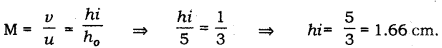# An object of height 5 cm is placed perpendicular to the principal axis of a concave lens of focal length 10 cm

An object of height 5 cm is placed perpendicular to the principal axis of a concave lens of focal length 10 cm. Use lens formula to determine the position, size and nature of the image if the distance of the object from the lens is 20 cm.

We have, height of object (hi) = 5 cm,
Focal length of lens (f) = -10 cm and,
Object distance (u) = -20 cmAgain magnificationThus, the image will be formed in front of the lens at a distance of 6.66 cm from the lens, virtual and erect of size 1.66 cm.